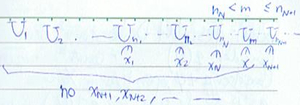# A Queue in $T_1$ Spaces

To find a finite subcover of a countable open cover $\left\{U_n\right\}_{n \in \N}$ of a $T_1$ space $X$ which has the Bolzano–Weierstrass property, one has to pick a sequence in $X$ in order to apply the Bolzano–Weierstrass property. In order to build a link between the sequence with the countable open cover, I picked a point $x_n$ from each $U_n$, and then build an infinite set $A$ consisting of $x_n \forall n \in \N$. I knew that $A$ is compact, but what does that do with the countable open cover?
Reading my notes again, I realised the trick of choosing $x_q$: it shouldn’t be chosen from $U_k$ with index $k$ which is smaller than $n_q$. In other words, $x_q \in U_{n_q}$ and $x_q \notin U_k \forall k < n_q$. If we failed to make such choices, that means we don't have enough $U_k$'s and $x_q$'s, meaning that $X$ is the finite union of $U_k$'s and it's countably compact. Otherwise, we continue this process to get an infinite set $A$ can be set up in the same way: $A := \left\{x_q \in X : q \in \N \right\}$. One will see that $A$ has no cluster point, contracdicting the Bolzano–Weierstrass property of $X$.
Assume that $x \in X$. $\exists m \in \N$ so that $x \in U_m$ and $x \notin U_j \forall j < m$. I was puzzled at the line $n_N < m \le n_{N + 1}$. Using the analogy of "queuing", I understood what's going on.
</source> </source>Since $X$ is $T_1$, which is equivalent to the closeness of singletons, deleting finitely many singletons from an open set won't change the openness of the set. We have a neighbourhood $V := U_m \setminus \left\{x_j : j \le N \text{ and } x \ne x_j \right\}$ of $x$ which intersects $A$ at no more than one point. Thus, there exists a deleted neighbourhood $V \setminus \{x\}$ of $x$ so that $V \cap A \setminus \{x\} = \varnothing$. Hence, $\forall x \in X, x \notin A'$.